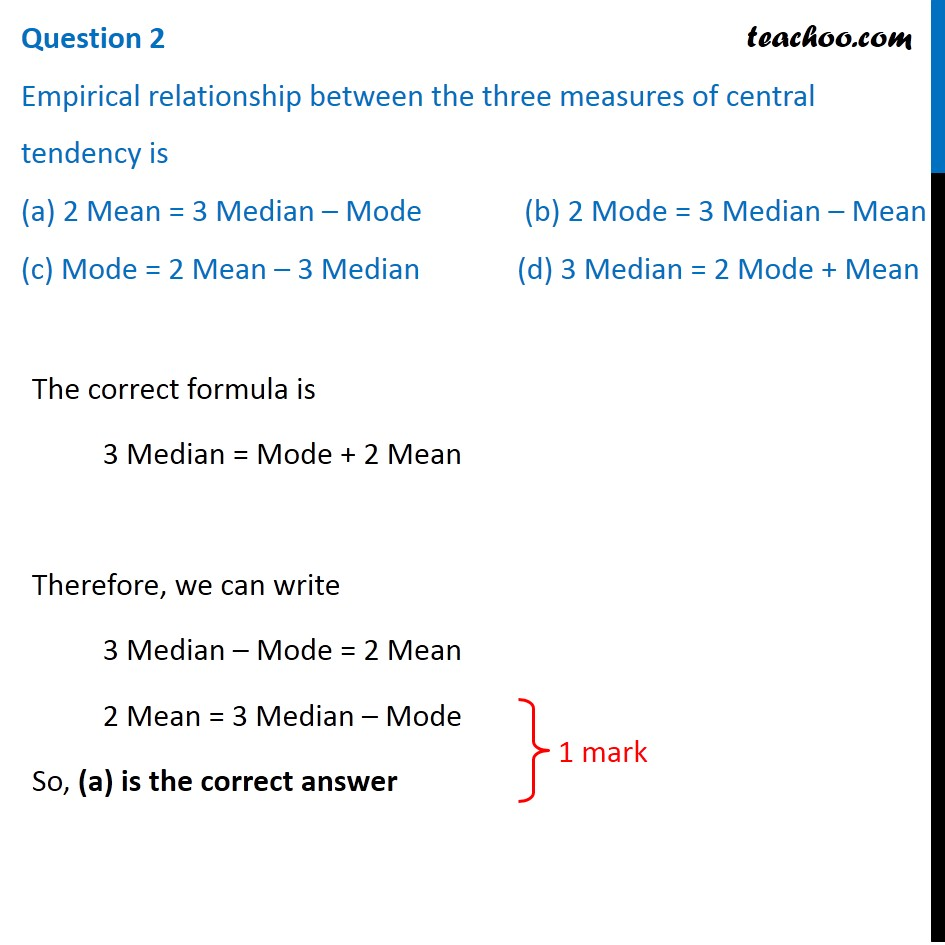## (c) Mode = 2 Mean – 3 Median         (d) 3 Median = 2 Mode + MeanSubscribe to our Youtube Channel - https://you.tube/teachoo

1. Class 10
2. Solutions of Sample Papers for Class 10 Boards
3. CBSE Class 10 Sample Paper for 2020 Boards - Maths Basic

Transcript

Question 2 Empirical relationship between the three measures of central tendency is (a) 2 Mean = 3 Median – Mode (b) 2 Mode = 3 Median – Mean (c) Mode = 2 Mean – 3 Median (d) 3 Median = 2 Mode + Mean The correct formula is 3 Median = Mode + 2 Mean Therefore, we can write 3 Median – Mode = 2 Mean 2 Mean = 3 Median – Mode So, (a) is the correct answer

CBSE Class 10 Sample Paper for 2020 Boards - Maths Basic

Class 10
Solutions of Sample Papers for Class 10 Boards